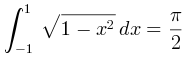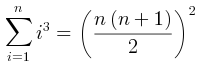Please provide math/equation rendering, it would be very useful to have for instance mathjax-rendered equations and maths in SVG diagrams.

+1 vote
answered Nov 25, 2016 by (257,860 points)
selected Nov 27, 2016 by saad

We've put online the beta version with JLatexMath.

The result is far better:

http://www.plantuml.com/plantuml/uml/SoWkIImgoKqioU3Ap2k9rpMCCrGeBYmgqJ3KhOWpqaofKB1LACZKD-9oICrB0Ia30000

@startmath
int_-1^1 sqrt(1-x^2)dx = pi/2
@endmathWe have integrated this with the Creole parser.

Example:

 @startuml
:$int_0^1f(x)dx$;
:$x^2+y_1+z_12^34$;
note right
Try also
$d/dxf(x)=lim_(h->0)(f(x+h)-f(x))/h$
end note
@enduml

http://www.plantuml.com/plantuml/uml/ixB9JIp9iClCAuatY3DCqwZGJAcmqGUBMNDPGIGhueoqA-CDjQlY3OtYZ4tWqddv9QaAHPdf6ILS8KML2eavnVbS41qf-YaL8BDiSp9pupKoTEqCD3M0VEqCJLsGiAP-1jGGhjIy50MGEG00

Tell us if you find issues.
You can also download last beta https://dl.dropboxusercontent.com/u/13064071/plantuml.jar and unzip the following file https://dl.dropboxusercontent.com/u/13064071/plantuml-jlatexmath.zip
(All .jar must be in the same folder)

+1 vote
answered Nov 16, 2016 by (257,860 points)
Mathjax looks javascript based, so integrating this with PlantUML (which is Java based) is not really possible.

If you find any OpenSource Java library that does the job (that is enabling math equation), we could considere integrating it.
commented Nov 17, 2016 by (120 points)
I'll do some research to see which may be more suitable in terms of ease of integration among the libs referenced in this URL:
http://stackoverflow.com/questions/456002/displaying-fancy-equations-with-java
+1 vote
answered Nov 17, 2016 by (257,860 points)
After having read the stackoverflow page, JEuclid may do the job, since it looks able to generated PNG/SVG images.
If someone find a better library, please post...

However, there are some points:

1) Last version of JEuclid was published in 2010 (so it is not active anymore)

2) JEuclid uses MathML. MathML is quite verbose : is it ok for you ? (See http://jeuclid.sourceforge.net/example5.html )

(although there is a ASL version of PlantUML). We could do the same trick as for PDF export : loading the library at runtime (see http://plantuml.com/pdf )

4) It would be nice is someone (you? :-) could make a snipset (simple code example) with jeuclid-minimal-3.1.9-distribution that shows how to use Jeuclide from Java code (that is transforming some XML data to PNG/SVG image).
+1 vote
answered Nov 18, 2016 by (257,860 points)

We were able to write a snipset that works for PNG with JEuclid.

For the record:

public static void main(String[] args) throws SAXException, IOException {

Parser parser = Parser.getInstance();
DocumentBuilder builder = parser.getDocumentBuilder();

String s = "<?xml version=\"1.0\"?><!DOCTYPE math PUBLIC \"-//W3C//DTD MathML 2.0//EN\" \"http://www.w3.org/TR/MathML2/dtd/mathml2.dtd\"><math mode=\"display\"><mrow><munderover><mo>&#x222B;</mo><mn>1</mn><mi>x</mi></munderover><mfrac><mi>dt</mi><mi>t</mi></mfrac></mrow>[/itex]";
InputStream is = new ByteArrayInputStream(s.getBytes());
Document doc = builder.parse(is);

Converter conv = Converter.getInstance();

LayoutContextImpl.getDefaultLayoutContext();
BufferedImage img = conv.render(doc, LayoutContextImpl.getDefaultLayoutContext());
ImageIO.write(img, "png", new File("out.png"));

}

Now this is working with MathML, and we still thinks that this is too verbose syntax.
Using AsciiMath syntax http://asciimath.org/ would probably be better.

There is a library ( https://webapps.ph.ed.ac.uk/snuggletex-mathplayground/asciimath-parser/ ) that can transform ASCIIMath to MathML so that would be perfect.
This library is written in JavaScript, but maybe we can run it using Nashorn/Rhino script engine, or translate it in pure Java.

commented Nov 19, 2016 by (120 points)
Thank you for the prompt response !
I totally agree with your argument on JEuclid, and the ASCIIMath->MathML solution looks promising for syntax simplicity and compatibility.
However, it seems inevitable to overcome the need for compatibility with javascript (e.g. Rhino). Which brings up the question, would MathJax be a possible solution, given that it offers syntax simplicity like ASCIIMath but with better rendering capability and extended math support. Do you think it's possible ?
answered Nov 25, 2016 by (257,860 points)

Starting wih V8050, we use AsciiMathParser.js (included in plantuml.jar) and external JEuclid libraries (here https://dl.dropboxusercontent.com/u/13064071/jeuclid.zip ).
It's not yet fully integrated with PlantUML (you cannot yet use it with diagrams).

We've created a new @startmath/@endmath to test the concept.
So you can have now:

@startmath
sum_(i=1)^n i^3=((n(n+1))/2)^2
@endmathhttp://www.plantuml.com/plantuml/uml/SoWkIImgoKqioU0gBisDryYqDTICorF8Z3EsrT38qyZJDjJKr3VIZ3FYSaZDIm6h0m00

If you want to try it with your own version of PlantUML, you can use V8050 and extract all libraries from https://dl.dropboxusercontent.com/u/13064071/jeuclid.zip in the same folder as plantuml.jar

The rendering is not as beautifull as https://www.mathjax.org/
Mathjax needs a browser and rhino is just a Javascript engine.
So I am not sure that Javascript engine can fully run mathjax.

If anymore successfully integrates Mathjax with Java, please let us known!

The next step will be to integrate this into our creole parser, so that we can have something like:

@startuml
Bob -> Alice : Can you calculate $sum_(i=1)^n i^3=((n(n+1))/2)^2$ ?
@enduml

But before doing that, we would like to know if the rendering is ok for you.
(We don't want to work on unused feature :-)

commented Nov 25, 2016 by (14,160 points)
For me, the left part of the png is truncated, not the svg
answered Nov 25, 2016 by (257,860 points)

Since the drawing with JEuclid is not perfect, we give a try with JLatexMath https://github.com/opencollab/jlatexmath
This project is very well documented.
We used http://www.intmath.com/help/asciimath-input-latex-katex-output.php to transform sum_(i=1)^n i^3=((n(n+1))/2)^2 into LaTeX

\\displaystyle{\\sum_{{{i}={1}}}^{{n}}}{i}^{3}={\\left(\\frac{{{n}{\\left({n}+{1}\\right)}}}{{2}}\\right)}^{2}

Using this LaTeX expression, we were able to generate the two files with JLatexMath:
https://dl.dropboxusercontent.com/u/13064071/example1.png
https://dl.dropboxusercontent.com/u/13064071/example1.svg

The result is really better.

Now we have to find a way to transform ASCIImath to LaTeX.
We found a library (JavaScript or PHP) that does the job:
https://github.com/asciimath/asciimathml/tree/master/asciimath-based

So either we used rhino again to intregrate this, or we port the PHP code to Java